# Multiplication Models Worksheets

Multiplication models reinforce the knowledge in understanding the multiplication concepts. The printable worksheets contain skills based on writing multiplication sentences, describing models, completing equations and drawing models. Equal groups, arrays, area and number lines are the four models used in the pdf worksheets for 2nd grade, 3rd grade, and 4th grade children. Explore some of them for free!

## Multiplication Model: Equal Groups

Describing Equal Groups

There are two models in each worksheet for grade 2 and grade 3. Answer the questions that describe each multiplication model. Real-life objects are used.Writing Multiplication Sentences

Write a multiplication sentence to describe each model. Level 1 has factors from 1 to 5. Level 2 has factors up to 12.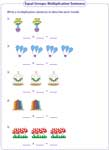Level 1:

Grab 'em All

Level 2:

Grab 'em All

Completing Multiplication Sentences

Each worksheet has five problems completing multiplication equation that describing a multiplication model.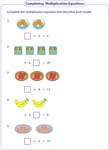Level 1:

Grab 'em All

Level 2:

Grab 'em All

Identifying Sentences; Drawing Groups

Each worksheet contains two sections. Section 1: Identifying multiplication sentences. Section 2: Drawing equal groups to match the multiplication sentences.Level 1:

Grab 'em All

Level 2:

Grab 'em All

## Multiplication Model: Arrays

Writing Multiplication Sentences

Find the number of rows and columns in each array. Write a multiplication sentence to describe each array.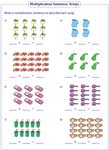Level 1:

Grab 'em All

Level 2:

Grab 'em All

Completing Multiplication Sentences

Complete the multiplication equation that describes each array.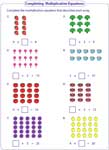Level 1:

Grab 'em All

Level 2:

Grab 'em All

Drawing Arrays

Draw an array to find the answer to each multiplication sentence.## Multiplication Model: Area

Writing Multiplication Sentences

Count the rows and columns of a rectangular region in each grid. Students of grade 3 and grade 4 need to write a multiplication sentence to describe each area model.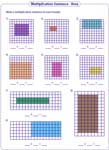Drawing Rectangular Regions

Draw a rectangular region in the grid to find the answer to each multiplication sentence in these printable worksheets.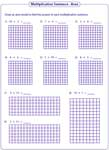Area Model: Mixed Review

Each worksheet is a combination of two types, writing multiplication sentence and drawing area model.## Multiplication Model: Number Lines

Writing Multiplication Sentences

Write a multiplication sentence that best describes the hops shown in each number line.Completing Multiplication Sentences

Each pdf worksheet has six problems completing multiplication equation using number line.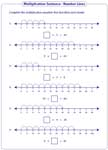Drawing Hops

Draw hops to show each multiplication sentence. Find an answer from your drawings.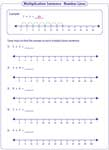Related Worksheets